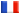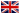# Séminaire de Cryptographie

## Hendrik Hubrechts### Point counting in families of hyperelliptic curves

Let p be a small prime number, F a field of characteristic p and extension degree n, and E a hyperelliptic curve over F. In cryptography one tries to exploit the hardness of determining a discrete logarithm on the jacobian of such curves. In order to achieve this it is important to know what the size of this jacobian is. This parameter can be deduced from the zeta function of the curve.

We will present algorithms to compute this zeta function for curves in one parameter families. The advantage of such `deformation' algorithms, when compared with Kedlaya's classical algorithm, is mainly a dramatically reduced memory usage, although a decrease in time requirements is attainable as well. We will also show the results of an implementation of such an algorithm.